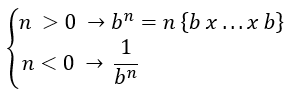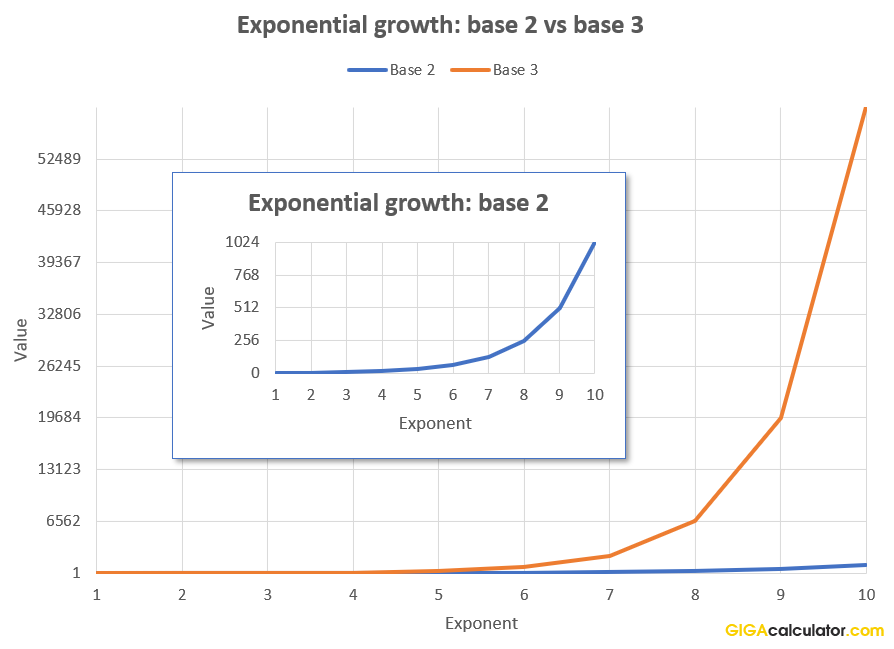# Exponent Calculator

Use this exponentiation calculator to easily calculate the power of a given base, raised by a given exponent. Use when faced with exponential growth, exponential increase or decrease scenarios.

Share calculator:

Embed this tool:
get code

## Exponentiation. How to calculate exponents?

Exponentiation is a mathematical operation in which a number called the base is raised to a power, which is given by the exponent. The exponent is usually written as a superscript, for example if the base is b and the exponent e the exponentiation of b by e will be be. This is the operation this online exponent calculator performs for you.

When the exponent is a positive integer the operation is equal to multiplying the base by itself e times. If the exponent is a negative, for example -5, the operation is 1 divided by the base raised to the absolute value of the exponent: b-5 = 1 / b5. The formula below expresses this. The most commonly computed exponents are the square of a number (b2, b raised to the second power / b to the power of two) and the cube of a number (b3, b raised to the 3-rd power / b to the power of 3), and their inverse functions: square root and cube root.

Exponents are related to logarithms so that the natural logarithm ln(e) is the inverse of the exponential function be, however defining exponentiation using logarithms is more common when dealing with complex numbers.

In combinatorics exponents express the number of possible permutations, that is - how many unique ways there are to select a part of a collection, or a set of things in which the order matters. You can use our permutation calculator for these calculations as well.

## Exponent formula

The formula for exponentiation is:where n is the exponent and b is the base. An exponent with base 0 does not technically exist, so it is not supported by our exponent calculator.

## Practical application of exponents

Exponentiation has uses in economics (e.g. compound interest), biology (population growth is often exponential), chemistry, physics, and, of course - computer science where storage capacity units are traditionally based off the powers of two, while public-key cryptographic functions rely on the hard reversibility of exponentiation. Practitioners of these disciplines often end up using an exponential calculator.

In physics we have exponential decay related to light, sound, gravity, dangerous chemicals, and radiation (see radioactive decay & half-life). In marketing and the spreading of ideas, memes, viral videos, etc. we talk about exponentially increasing reach. Moore's law about the increase of computing power is in essence an exponential law. The scale for measuring Earthquakes is also exponential, so a magnitude 5 earthquake is 32 times stronger than a magnitude 4 earthquake (101.5), while a magnitude 6 earthquake releases 1,000 times more energy (103) than a 4. The decibel scale used to measure volume is also exponential: 20 dB = 102 dB = 100 power ratio, 30 dB = 103 = 1,000 power ratio, so a 30 dB sound level is 10 times louder than a 20 dB sound level.In the graph above, you can think of each exponent as a generation (in biology), or as a tier of people who have seen a viral video or read a viral article (in marketing), or the power released from an earthquake of a magnitude equal to the exponent. As you can see by step 10 an exponential process that started with a base of 2 is completely dwarfed by one started with a base of 3 to such an extent that the base 2 line looks almost like a flat line next to the base 3 exponential curve, which is why we had to provide a zoom-in. The graph was compiled using our online tool.

In complex systems exponential processes often precede cataclysmic events, such as ruptures and explosions. The distribution of work contribution, ability / talent, and wealth in humans and other species is exponential according to work  often cited by the famous psychometrician Jordan B. Peterson. In the human studies circles it is known as the Paretian distribution (Pareto distribution).

Financial crises, economic crises and bubbles have exponential nature before they pop according to Didier Sornette & colleagues . The size of cities is well predicted using power law (Zipf's Law)  which follows an exponential function.